Search

Conics: Ellipses:
Finding Information from the Equation
(page 2 of 4)

Sections: Introduction, Finding information from the equation, Finding the equation from information, Word ProblemsYour first task will usually be to demonstrate that you can extract information about an ellipse from its equation, and also to graph a few ellipses.

• State the center, vertices, foci and eccentricity of the ellipse with general
equation
16x2 + 25y2 = 400, and sketch the ellipse.
• To be able to read any information from this equation, I'll need to rearrange it to get "=1", so I'll divide through by 400. This gives me: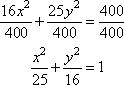Since x2 = (x  0)2 and y2 = (y  0)2, the equation above is really: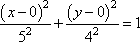Then the center is at (h, k) = (0, 0). I know that the a2 is always the larger denominator (and b2 is the smaller denominator), and this larger denominator is under the variable that parallels the longer direction of the ellipse. Since 25 is larger than 16, then a2 = 25, a = 5, and this ellipse is wider (paralleling the x-axis) than it is tall. The value of a also tells me that the vertices are five units to either side of the center, at (5, 0) and (5, 0).

To find the foci, I need to find the value of c. From the equation, I already have a2 and b2, so:

a2  c2 = b2
25  c2 = 16

9 = c2

Then the value of c is 3, and the foci are three units to either side of the center, at (3, 0) and (3, 0). Also, the value of the eccentricity e is c/a = 3/5.

 To sketch the ellipse, I first draw the dots for the center and the endpoints of each axis: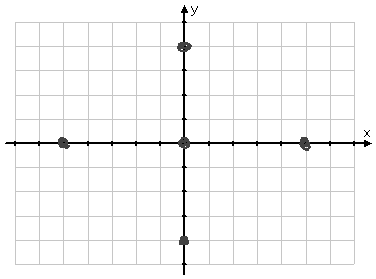Then I rough in a curvy line, rotating my paper as I go and eye-balling my curve for smoothness...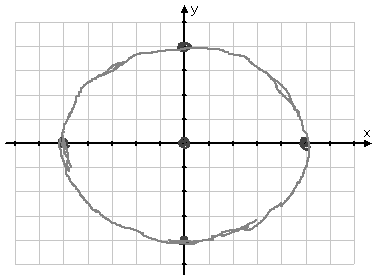...and then I draw my "answer" as a heavier solid line.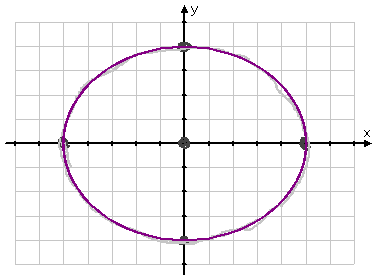center (0, 0), vertices (5, 0) and (5, 0), foci (3, 0) and (3, 0), and eccentricity 3/5

You may find it helpful to do the roughing in with pencil, rotating the paper as you go around, and then draw your final graph in pen, carefully erasing your "rough draft" before you hand in your work. And always make sure your graph is neat and is large enough to be clear.

• State the center, foci, vertices, and co-vertices of the ellipse with equation
25x2 + 4y2 + 100x  40y + 100 = 0. Also state the lengths of the two axes.
• I first have to rearrange this equation into conics form by completing the square and dividing through to get "=1". Once I've done that, I can read off the information I need from the equation.

25x2 + 4y2 + 100x  40y = 100
25x2 + 100x + 4y2  40y = 100

25(x2 + 4x       ) + 4(y2  10y        ) = 100 + 25(    ) + 4(     )

25(x2 + 4x + 4) + 4(y2  10y + 25) = 100 + 25( 4 ) + 4( 25 )

25(x + 2)2 + 4(y  5)2 = 100 + 100 + 100 = 100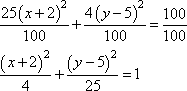The larger demoninator is a2, and the y part of the equation has the larger denominator, so this ellipse will be taller than wide (to parallel the y-axis). Also, a2 = 25 and b2 = 4, so the equation b2 + c2 = a2 gives me 4 + c2 = 25, and c2 must equal 21. The center is clearly at the point (h, k) = (2, 5). The vertices are a = 5 units above and below the center, at (2, 0) and (2, 10). The co-vertices are b = 2 units to either side of the center, at (4, 5) and (0, 5). The major axis has length 2a = 10, and the minor axis has length 2b = 4. The foci are messy: they're sqrt units above and below the center.

center (2, 5), vertices (2, 0) and (2, 10),
co-vertices
(4, 5) and (0, 5), foci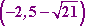and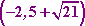,
major axis length
10, minor axis length 4

<< Previous  Top  |  1 | 2 | 3 | 4  |  Return to Index  Next >>

 Cite this article as: Stapel, Elizabeth. "Conics: Ellipses: Finding Information from the Equation." Purplemath.     Available from https://www.purplemath.com/modules/ellipse2.htm.     Accessed [Date] [Month] 2016

Study Skills Survey

Tutoring from Purplemath
Find a local math tutor